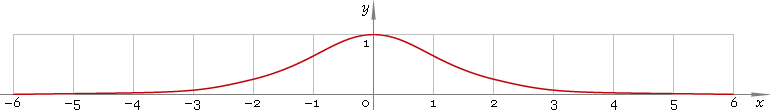The Art of Mathematics

# sech — hyperbolic secant function

## 1. Definition

Hyperbolic secant is defined as

sechx ≡ 2 /(ex + ex)

## 2. Graph

Hyperbolic secant is symmetric function defined everywhere on real axis. Its graph is depicted below — fig. 1.Fig. 1. Graph of the hyperbolic secant function y = sechx.

Function codomain is limited to the range (0, 1].

## 3. Identities

Base:

sech2x + tanh2x = 1

By definition:

sechx ≡ 1 /coshx

Property of symmetry:

sech−x = sechx

## 4. Derivative and indefinite integral

Hyperbolic secant derivative:

sech′x = −sechx tanhx ≡ sinhx /cosh2x

Indefinite integral of the hyperbolic secant:

∫ sechx dx = arctan(sinhx) + C

where C is an arbitrary constant.

## 5. How to use

To calculate hyperbolic secant of the number:

``sech(−1);``

To get hyperbolic secant of the complex number:

``sech(−1+i);``

To get hyperbolic secant of the current result:

``sech(rslt);``

To get hyperbolic secant of the number z in calculator memory:

``sech(mem[z]);``

## 6. Support

Hyperbolic secant of the real argument is supported in free version of the Librow calculator.

Hyperbolic secant of the complex argument is supported in professional version of the Librow calculator.